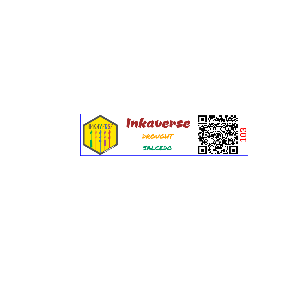# Create fieldbook

The fieldbook experimental design was deployed with `inti` package https://inkaverse.com/articles/apps.html

``````library(inti)

treats <- data.frame(condition = c("irrigated", "drought")
, genotypes = c("choclito", "salcedo", "pandela", "puno"))

design <- tarpuy_design(data = treats
, nfactors = 2
, type = "rcbd"
, rep = 3
, barcode = "HUITO")

fb <- design\$fieldbook

fb %>% web_table()``````

# Label design

``````library(huito)

font <- c('Ceviche One', "Permanent Marker")

huito_fonts(font)

label <- fb %>%
label_layout(size = c(10, 2.5)
, border_color = "blue"
) %>%
include_image(
value = "https://flavjack.github.io/inti/img/inkaverse.png"
, size = c(2.4, 2.4)
, position = c(1.2, 1.25)
) %>%
include_barcode(
value = "barcode"
, size = c(2.5, 2.5)
, position = c(8.2, 1.25)
) %>%
include_text(value = "plots"
, position = c(9.7, 1.25)
, angle = 90
, size = 15
, color = "red"
) %>%
include_text(value = "Inkaverse"
, position = c(4.6, 2)
, size = 30
, color = "brown"
, font
) %>%
include_text(value = "condition"
, position = c(4.6, 1.2)
, size = 13
, color = "orange"
, font
) %>%
include_text(value = "genotypes"
, position = c(4.6, 0.5)
, size = 13
, color = "#009966"
, font
) ``````

After running the chunk the first time. You should comment `huito_fonts()` as we already imported the fonts.

## Preview mode

The preview mode `label_print(mode = "preview")` generate a example of the label design from a random row of the data set.

``````label %>%
label_print(mode = "preview")``````## Complete mode

If you want generate the complete labels list, change: `label_print(mode = "complete")`.

``````label %>%
label_print(mode = "complete", filename = "etiquetas", nlabels = 10)``````